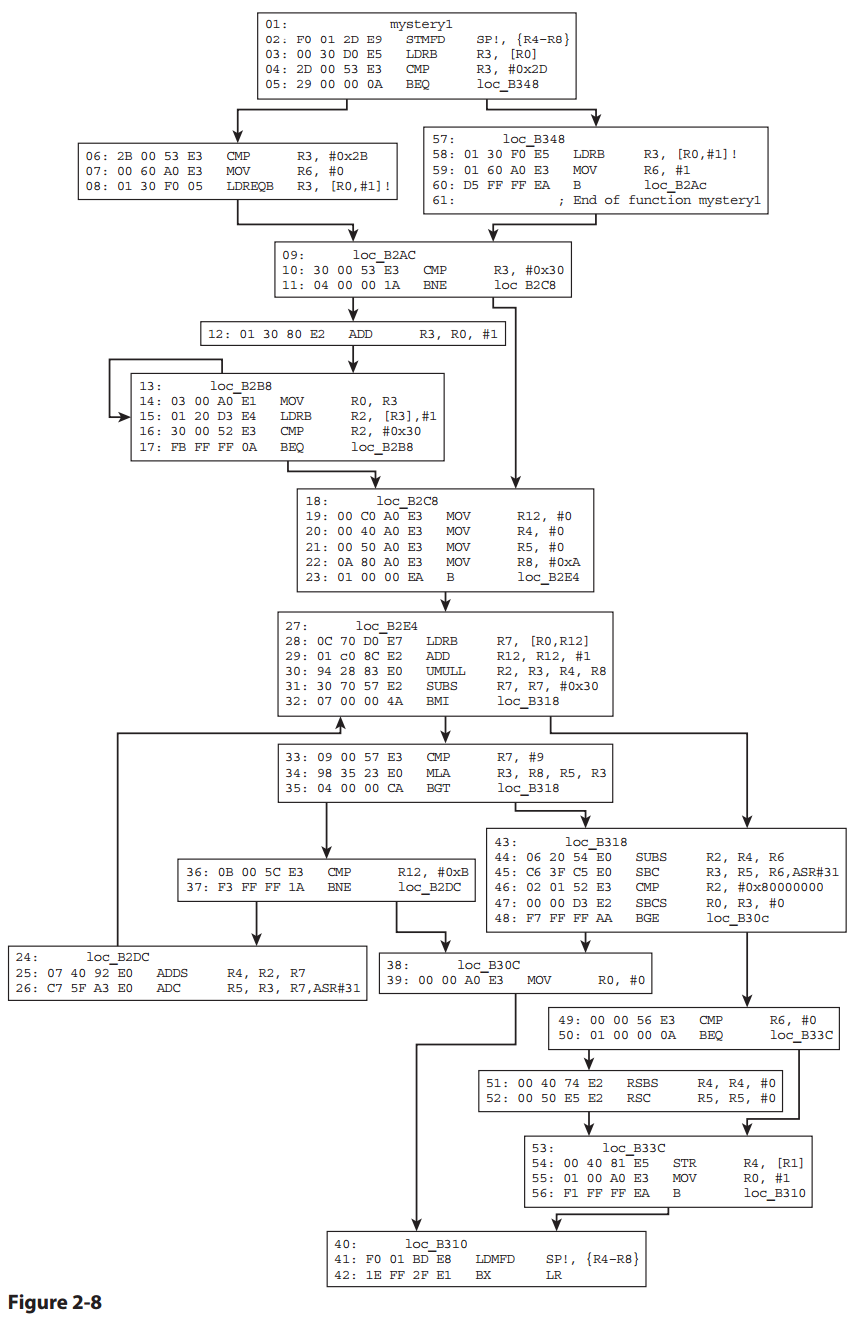## Monday, January 12, 2015

### Practical Reverse Engineering p. 78 #1

Question number 1 on page 78 of Practical Reverse Engineering is as follows:

Figure 2-8 shows a function that takes two arguments. It may seem somewhat challenging at fi rst, but its functionality is very common. Have patience.

Indeed the disassembly does appear to be a reasonably complex function.Figure 2-8. Practical Reverse Engineering. © 2014 by Bruce Dang

This function is not in Thumb state as every instruction is 32-bits in width. It attempts to convert an ASCII string to a signed decimal number. It is very lenient as far as input (even accepting non-numerical characters in the string input), however it will not work on strings exceeding the MAX_INT constant of 2147483648 (0x80000000).

Here is a close 1 to 1 approximation from ARM to C:

```BOOL ascii_to_decimal(const char *str, int32_t *retNum)
{
BOOL negative;
int i = 0;              /* LDRB R3, [R0] */

/* CMP R3, #0x2D */
if  (str[i] == '-')
{
++i;                /* LDRB R3, [R0,#1]! */
negative = TRUE;    /* MOV R6, #1 */
}
else
{
negative = FALSE;   /* MOV R6, #0 */

/* CMP R3, #0x2B */
if (str[i] == '+')
++i;            /* LDREQB R3, [R0,#1]! */
}

/* CMP R3, #0x30 */
if (str[i] == '0')
{
/* CMP R2, #0x30 */
while (str[i] == '0')
++i;            /* LDRB R2, [R3],#1 */
}

const int base = 10;    /* MOV R8, #0xA */
int64_t result = 0;

while (TRUE)
{
result *= base;         /* UMULL R2, R3, R4, R8 */
result += str[i] - '0'; /* ADDS R4, R2, R7 */
++i;                    /* ADD R12, R12, #1 */

/* SUBS R7, R7, #0x30 */
if (str[i] < '0')
break;

/* CMP R7, #9 */
if (str[i] > '9')
break;
}

/* CMP R2, #0x80000000 */
if (abs((int32_t) result) > INT_MAX)
return FALSE;               /* MOV R0, #0 */

*retNum = (int32_t) result;     /* STR R4, [R1] */
return TRUE;                    /* MOV R0, #1 */
}
```

#### 1 comment :

1.Thanks for your series of blog posts, they are very helpful to my own exercise solving attempts. I am slightly irritated by the following sequence of instructions:

44: 06 20 54 E0 SUBS R2, R4, R6
45: C6 3F C5 E0 SBC R3, R5, R6,ASR#31
46: 02 01 52 E3 CMP R2, #0x80000000
47: 00 00 D3 E2 SBCS R0, R3, #0
48: F7 FF FF AA BGE loc_B30c

Do you know why there are two instructions after another (line 46 and 47) modifying the conditional flags of CPSR? Shouldn't the BGE instruction come immediately after the CMP instruction?